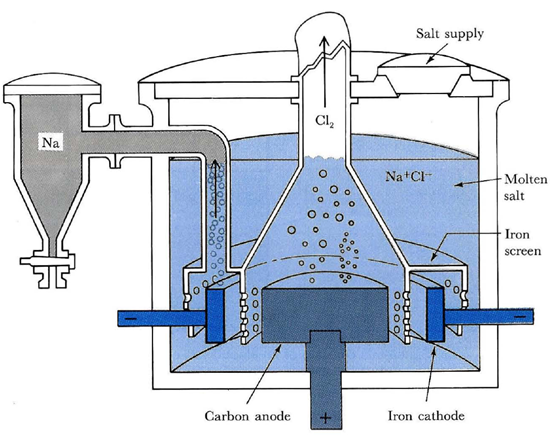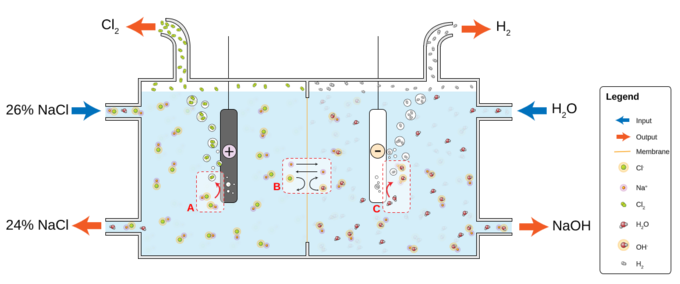## Electrolysis of Sodium Chloride

#### Learning Objective

• Predict the products of electrolysis of sodium chloride under molten and aqueous conditions

#### Key Points

• Sodium metal and chlorine gas can be obtained with the electrolysis of molten sodium chloride.
• Electrolysis of aqueous sodium chloride yields hydrogen and chlorine, with aqueous sodium hydroxide remaining in solution.
• The reason for the difference is that the reduction of Na+ (E° = –2.7 v) is energetically more difficult than the reduction of water (–1.23 v).

#### Terms

• anodeThe electrode of an electrochemical cell at which oxidation occurs.
• cathodeThe electrode of an electrochemical cell at which reduction occurs.

## Electrolysis of NaCl

As we have covered, electrolysis is the passage of a direct electric current through an ionic substance that is either molten or dissolved in a suitable solvent. This results in chemical reactions at the electrodes and the separation of materials. Two commonly used methods of electrolysis involve molten sodium chloride and aqueous sodium chloride. You might think that both methods would give you the same products, but this not the case. Let’s go through each of the methods to understand the different processes.

## Electrolysis of Molten NaCl

If sodium chloride is melted (above 801 °C), two electrodes are inserted into the melt, and an electric current is passed through the molten salt, then chemical reactions take place at the electrodes.Electrolysis cell for molten sodium chlorideA commercial electrolysis cell for the production of metallic sodium and chlorine gas from molten NaCl. Liquid sodium floats to the top of the melt above the cathode and is drained off into a storage tank. Chlorine gas bubbles out of the melt above the anode.

Sodium ions migrate to the cathode, where electrons enter the melt and are reduced to sodium metal:

${Na}^{+} + {e}^{-} \rightarrow Na$

Chloride ions migrate the other way, toward the anode. They give up their electrons to the anode and are oxidized to chlorine gas:

${Cl}^{-} \rightarrow \frac{1}{2}{Cl}_{2} + {e}^{-}$

The overall reaction is the breakdown of sodium chloride into its elements:

$2NaCl \rightarrow 2Na(s) + {Cl}_{2}(g)$

## Electrolysis of Aqueous NaCl

What happens when we have an aqueous solution of sodium chloride? Well, we can’t forget that we have to factor water into the equation. Since water can be both oxidized and reduced, it competes with the dissolved Na+ and Cl ions. Rather than producing sodium, hydrogen is produced.Electrolysis of aqueous sodium chlorideElectrolysis of aqueous NaCl results in hydrogen and chloride gas. At the anode (A), chloride (Cl-) is oxidized to chlorine. The ion-selective membrane (B) allows the counterion Na+ to freely flow across, but prevents anions such as hydroxide (OH-) and chloride from diffusing across. At the cathode (C), water is reduced to hydroxide and hydrogen gas. The net process is the electrolysis of an aqueous solution of NaCl into industrially useful products sodium hydroxide (NaOH) and chlorine gas.

The reaction at the cathode is:

${H}_{2}O (l) + 2 {e}^{- } \rightarrow {H}_{2}(g) + 2{ OH}^{- }$

The reaction at the anode is:

${Cl}^{- } \rightarrow \frac{1}{2} {Cl}_{2}(g) +1e^-$

The overall reaction is as follows:

$NaCl(aq) + {H}_{2}O(l) \rightarrow {Na}^{+}(aq) + {OH}^{-}(aq) + {H}_{2}(g) + \frac{1}{2}{Cl}_{2}(g)$

Reduction of Na+ (E° = –2.7 v) is energetically more difficult than the reduction of water (–1.23 v), so in aqueous solution, the latter will prevail.